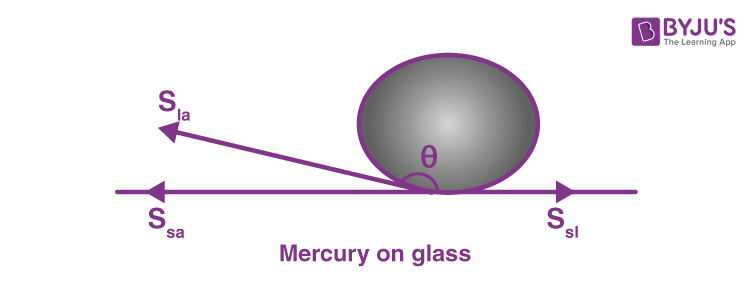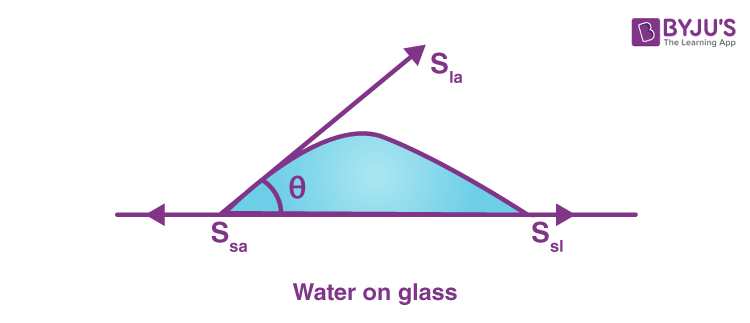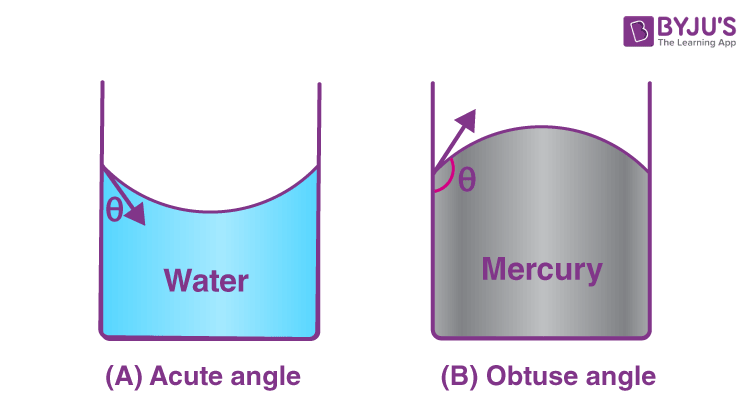# Relation Between Angle Of Contact And Temperature

We know that temperature is the measure of the degree of hotness or coldness of the body. It is measured in Celsius, Fahrenheit, and Kelvin scales. Temperature is affected by various factors like external environment, angle of contact, surface tension and many more factors. We are also aware that the angle of contact is inversely proportional to temperature. Let us know more about the angle of contact and its relationship with temperature.

## Angle Of Contact

The angle of contact concept can be witnessed when a liquid comes in contact with the solid. The adhesive and cohesive properties of the liquid determine the angle of contact. Adhesive force is the force of attraction between the molecules of two different materials. The force between the molecules of the same objects is known as a cohesive force.

The angle of contact determines if a liquid will spread on the surface of a solid or form droplets on it. The angle of contact is defined as the angle between the tangent to the liquid surface at the point of contact and the solid surface inside the liquid. It is represented as $\theta$.

The surface of liquid near the plane of contact with another medium is curved. Angle of contact is different for different pairs of solids and liquids interfaces. Angle of contact is determined by the θ value.Figure (a)Figure (b)

The above figure (a) represents the shape of the water drop on the lotus leaf, and figure (b) represents the shape of the water drop on the plastic plate.

NOTE:

• Droplet will be formed when the angle of contact is obtuse.
• Water will spread if the angle of contact is acute.

As per the figure above, we can witness interfacial tensions like

• Liquid-air tension denoted by Sla
• Solid-air tension denoted by Ssa
• Solid-liquid tension denoted by Ssl

At the line of contact, the surface forces between the three media must be in equilibrium.

Sla cos θ + Ssl = Ssa

If the angle of contact is an obtuse angle, then Ssl > Sla.

If the angle of contact is an acute angle, then Ssl < Sla.

When θ is an obtuse angle, then molecules of liquids are attracted strongly to themselves and weakly to those of solid.

Now let us know the relation between the angle of contact and temperature.

## Temperature and Angle of Contact

As we know, the adhesive and cohesive properties of the liquid are the key factors for the angle of contact. Surface tension causes an increase in cohesive forces. So when the temperature rises, surface tension decreases and the angle of contact increases.

Let us learn with an example about the relationship between the angle of contact and temperature.

Consider liquid inside a transparent vessel. Observing the surface point of contact of the liquid, you can make out that the contact point of the liquid is not exactly $90^{0}$.

The angle between the surface of the liquid and the outline of the contact surface defines the contact angle. The contact angle is a surface phenomenon.

For different solid-liquid interfacial tensions, the surface point varies and hence the contact point of the liquid also varies. It varies because of the adhesive and cohesive forces associated with the liquid and solids.

Adhesive force is seen between the molecules of different molecules. Here the adhesive force can be seen between the water molecules and the edge of the glass. The force between molecules of the same object is known as a cohesive force.

If the adhesive force is greater than the cohesive force, the contact angle is less than $90^{0}$. This can be seen between water and glass surfaces.

If the adhesive force is less than the cohesive force, the contact angle is greater than $90^{0}$. This can be seen between mercury and glass surfaces.

This can be illustrated by the figure below.The angle of contact varies from $0^{0}$ to $180^{0}$.

When the temperature increases, the cohesive force decreases. It happens because the energy associated with the material is high, which is contributed by the vibration of the particles. Here the contact angle decreases.

When the temperature decreases, the cohesive force increases. Hence, the contact angle increases.

Hope you understood the relation between angle of contact and temperature in a better way.

Stay tuned with BYJU’S for more such interesting topics in physics, chemistry and maths in an engaging way with video explanations.

## Frequently Asked Questions – FAQs

### What is contact angle?

The angle between the surface of the liquid and the outline of the contact surface is known as the contact angle.

### When the temperature decreases, what happens to the contact angle?

The contact angle increases.

### What happens to the cohesive force when the temperature increases?

The cohesive force decreases.

### When will a droplet be formed?

Droplet is formed when the angle of contact is obtuse.